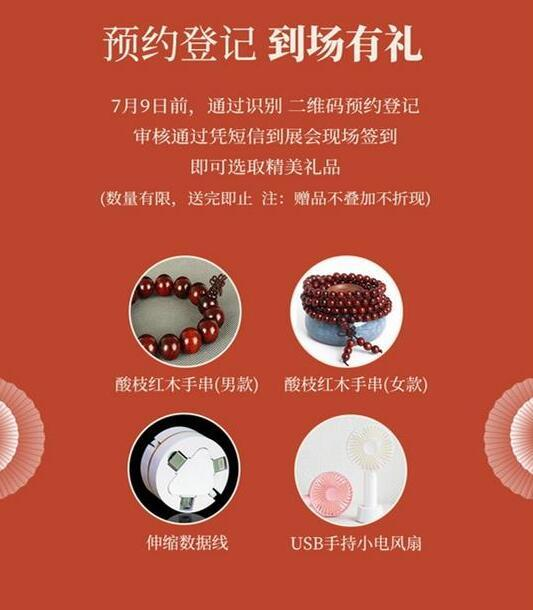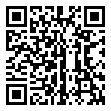|

# 第三届新中式红木家具展最新最全【逛展指南】出炉!

7月9日-14日，以“国潮中式 优选生活“为主题的第三届中国（中山）新中式红木家具展暨优选中式红木家具采购节（以下简称第三届新中式红木家具展）将在广东中山大涌红博城会展中心开幕。第三届中国（中山）新中式红木家具展暨优选中式红木家具采购节将在广东中山大涌红博城会展中心开幕

本次展会内容丰富多样，亮点十足，不仅有数十家红木活力品牌参展，还将举行第三届中国新中式红木家具展论坛、红木家具优秀品牌发布会、“新意思”新中式红木家具设计展、“匠心中式”红木家具精品评选、《艺术红木家具》标准修订启动仪式等活动。

这么多精彩纷呈的活动席卷而来，如果还没做功课，不用着急，离开展还有几天时间，是时候打开这份逛展指南，助你逛好新中式红木家具展。

1、展会时间：7月9日-14日

2、展会地点：广东中山大涌红博城会展中心（北广场）

3、展区分布：

本次展会共有数十家红木活力品牌参展，不妨先留意一下自己感兴趣的品牌展位：

新中式品牌综合展馆：国方家居（A01）、东成红木（A02a）、红古轩（A02b）、雅宋红木（A03）、忆古轩（A04）、玖月（A05）、墨+（A06）、印巷森刻（A07）、禾木上品（A08）、金隆红木（A09）、无名红木（A10）、朗州（A13）、唐明居（A14）、祥瑞坊（A15）、水雨轩（A16）、古木印象（A17）、伟世鸿泰（A18）、地天泰（A19）、方兴红木（A20）、永恒居（A21）、大富（A22a）、瀚典（A22b）、顺泰轩（A23）、匠王红木（A24）、华钦红木（A25）、国福干燥（A26）、建国红木（A27）

“新意思”新中式红木家具设计展区：中央民族大学、华南农业大学、中南林业科技大学、五邑大学、中山职业技术学院

“匠心中式”红木家具精品评选展区：雍博堂、大为之家、飞龙红木、善云红木、良辰轩、呈麟红木……第三届新中式红木家具展平面图

4、活动精彩纷呈亮点爆棚

作为业内知名的新中式红木家具展示交易平台，为更好的凸显当代红木家具的文化内涵和艺术魅力，本次展会策划了批发价买红木、名家精品论坛、“新意思”新中式红木家具设计展等亮点活动。

批发价买红木，全场价格低低低！超值折扣，批发价买红木

与经销商一起批发价买红木，实力打造省钱主场，是今年新中式红木展的贴心之处。消费者跟着更懂红木家具的经销商，可以用更低的价格买到高质量产品，同时，大大减低购入次货几率，更好地享受省心、省力的新时代生活方式。预约团购，到场有礼

7月9日前通过二维码预约，凭审核通过信息到现场签到即可抽取精美红木礼品，还可以在线领取现金抵用券，现场下单可直接抵作家具货款，满10万抵2000元，满5万抵1000元，满3万抵500元。转发集赞，赢“网红”豪礼

7月9日前转发新中式红木展宣传海报或邀请函到朋友圈，7月14日前到展会现场凭点赞截图即可领取一份精美红木礼品，包括128个赞获得萌宠移动电源，268个赞获得故宫口红（6支装）一套。

展会正式官宣，实力大咖重磅来袭！实力大咖重磅来袭

作为新中式红木展的系列主题活动，新中式家具发展论坛将邀请业界资深设计名家或者在专业领域内有独到见解的设计师，以“主题论坛”携手推动中式家居设计的交流发展，解读中式家居之美。

校企联手，这个设计展“有点意思“新意思”新中式红木家具设计展集结新锐中式设计

“新意思”新中式红木家具设计展是一个呈现优秀原创设计作品、搭建设计交流平台的家具设计展。2019年将再度联手国内知名高校与品牌企业，从不同的设计视角、不同的美感体验，呈现不同的新锐中式设计作品，彰显年轻设计师的锋芒与激情。

5、活动流程一览6、观众预约登记

看到这么多活动，先别急着手忙脚乱，点击进入新中式红木家具展官网，进行观众预约登记，登记成功便可在展会现场免费领取礼品！第三届新中式红木家具展观众预约登记流程

7、交通规划

①自驾：

可在高德地图、百度地图等地图软件上搜索“广东省中山市大涌红博城会展中心”。详细地址：位于中山市大涌镇歧涌路（大涌牌坊前进200米）处。

②市内公交路线：

中山汽车总站乘坐010路（大涌红博城站下车）

城南客运站乘坐057路（大涌红博城站下车）

城轨中山北站乘坐红木旅游专线K06路（大涌红博城站下车）

③高铁：

各地高铁站→广州南站

广州南站→城轨中山北站（行车时间大概40分钟）

城轨中山北站→乘坐的士或市内公交前往红博城

④机场：

距珠海三灶国际机场约75公里，抵达机场后，可乘坐机场班车、的士等公交工具抵达中山。

距广州白云机场约115公里，抵达机场后可乘坐机场班车、的士、高铁抵达中山。

珠海三灶国际机场班车时刻表：08:30-20:30（班次间隔：30分钟）

广州白云机场班车时刻表：08:30-22:50（班次间隔：1小时）大涌红博城会展中心

8、天气情况7月9日-14日中山市天气预报

7月9日-14日，中山以阴天、小雨为主，平均气温28-31℃，请您依据个人需要佩带雨具。

大涌美酒红木香，展会盛来琥珀光。

拿好这份逛展指南，开启您的展会之旅吧！7月9日-14日，我们在第三届新中式红木家具展等待您的到来。

`声明：本文由入驻焦点开放平台的作者撰写，除焦点官方账号外，观点仅代表作者本人，不代表焦点立场错误信息举报电话： 400-099-0099，邮箱：jubao@vip.sohu.com，或点此进行意见反馈，或点此进行举报投诉。`A B C D E F G H J K L M N P Q R S T W X Y Z
A - B - C - D - E
• A
• 鞍山
• 安庆
• 安阳
• 安顺
• 安康
• 澳门
• B
• 北京
• 保定
• 包头
• 巴彦淖尔
• 本溪
• 蚌埠
• 亳州
• 滨州
• 北海
• 百色
• 巴中
• 毕节
• 保山
• 宝鸡
• 白银
• 巴州
• C
• 承德
• 沧州
• 长治
• 赤峰
• 朝阳
• 长春
• 常州
• 滁州
• 池州
• 长沙
• 常德
• 郴州
• 潮州
• 崇左
• 重庆
• 成都
• 楚雄
• 昌都
• 慈溪
• 常熟
• D
• 大同
• 大连
• 丹东
• 大庆
• 东营
• 德州
• 东莞
• 德阳
• 达州
• 大理
• 德宏
• 定西
• 儋州
• 东平
• E
• 鄂尔多斯
• 鄂州
• 恩施
F - G - H - I - J
• F
• 抚顺
• 阜新
• 阜阳
• 福州
• 抚州
• 佛山
• 防城港
• G
• 赣州
• 广州
• 桂林
• 贵港
• 广元
• 广安
• 贵阳
• 固原
• H
• 邯郸
• 衡水
• 呼和浩特
• 呼伦贝尔
• 葫芦岛
• 哈尔滨
• 黑河
• 淮安
• 杭州
• 湖州
• 合肥
• 淮南
• 淮北
• 黄山
• 菏泽
• 鹤壁
• 黄石
• 黄冈
• 衡阳
• 怀化
• 惠州
• 河源
• 贺州
• 河池
• 海口
• 红河
• 汉中
• 海东
• I
• J
• 晋中
• 锦州
• 吉林
• 鸡西
• 佳木斯
• 嘉兴
• 金华
• 景德镇
• 九江
• 吉安
• 济南
• 济宁
• 焦作
• 荆门
• 荆州
• 江门
• 揭阳
• 金昌
• 酒泉
• 嘉峪关
K - L - M - N - P
• K
• 开封
• 昆明
• 昆山
• L
• 廊坊
• 临汾
• 辽阳
• 连云港
• 丽水
• 六安
• 龙岩
• 莱芜
• 临沂
• 聊城
• 洛阳
• 漯河
• 娄底
• 柳州
• 来宾
• 泸州
• 乐山
• 六盘水
• 丽江
• 临沧
• 拉萨
• 林芝
• 兰州
• 陇南
• M
• 牡丹江
• 马鞍山
• 茂名
• 梅州
• 绵阳
• 眉山
• N
• 南京
• 南通
• 宁波
• 南平
• 宁德
• 南昌
• 南阳
• 南宁
• 内江
• 南充
• P
• 盘锦
• 莆田
• 平顶山
• 濮阳
• 攀枝花
• 普洱
• 平凉
Q - R - S - T - W
• Q
• 秦皇岛
• 齐齐哈尔
• 衢州
• 泉州
• 青岛
• 清远
• 钦州
• 黔南
• 曲靖
• 庆阳
• R
• 日照
• 日喀则
• S
• 石家庄
• 沈阳
• 双鸭山
• 绥化
• 上海
• 苏州
• 宿迁
• 绍兴
• 宿州
• 三明
• 上饶
• 三门峡
• 商丘
• 十堰
• 随州
• 邵阳
• 韶关
• 深圳
• 汕头
• 汕尾
• 三亚
• 三沙
• 遂宁
• 山南
• 商洛
• 石嘴山
• T
• 天津
• 唐山
• 太原
• 通辽
• 铁岭
• 泰州
• 台州
• 铜陵
• 泰安
• 铜仁
• 铜川
• 天水
• 天门
• W
• 乌海
• 乌兰察布
• 无锡
• 温州
• 芜湖
• 潍坊
• 威海
• 武汉
• 梧州
• 渭南
• 武威
• 吴忠
• 乌鲁木齐
X - Y - Z
• X
• 邢台
• 徐州
• 宣城
• 厦门
• 新乡
• 许昌
• 信阳
• 襄阳
• 孝感
• 咸宁
• 湘潭
• 湘西
• 西双版纳
• 西安
• 咸阳
• 西宁
• 仙桃
• 西昌
• Y
• 运城
• 营口
• 盐城
• 扬州
• 鹰潭
• 宜春
• 烟台
• 宜昌
• 岳阳
• 益阳
• 永州
• 阳江
• 云浮
• 玉林
• 宜宾
• 雅安
• 玉溪
• 延安
• 榆林
• 银川
• Z
• 张家口
• 镇江
• 舟山
• 漳州
• 淄博
• 枣庄
• 郑州
• 周口
• 驻马店
• 株洲
• 张家界
• 珠海
• 湛江
• 肇庆
• 中山
• 自贡
• 资阳
• 遵义
• 昭通
• 张掖
• 中卫

1室1厅1厨1卫1阳台

1
2
3
4
5

0
1
2

1

1

0
1
2
3报名成功，资料已提交审核A B C D E F G H J K L M N P Q R S T W X Y Z
A - B - C - D - E
• A
• 鞍山
• 安庆
• 安阳
• 安顺
• 安康
• 澳门
• B
• 北京
• 保定
• 包头
• 巴彦淖尔
• 本溪
• 蚌埠
• 亳州
• 滨州
• 北海
• 百色
• 巴中
• 毕节
• 保山
• 宝鸡
• 白银
• 巴州
• C
• 承德
• 沧州
• 长治
• 赤峰
• 朝阳
• 长春
• 常州
• 滁州
• 池州
• 长沙
• 常德
• 郴州
• 潮州
• 崇左
• 重庆
• 成都
• 楚雄
• 昌都
• 慈溪
• 常熟
• D
• 大同
• 大连
• 丹东
• 大庆
• 东营
• 德州
• 东莞
• 德阳
• 达州
• 大理
• 德宏
• 定西
• 儋州
• 东平
• E
• 鄂尔多斯
• 鄂州
• 恩施
F - G - H - I - J
• F
• 抚顺
• 阜新
• 阜阳
• 福州
• 抚州
• 佛山
• 防城港
• G
• 赣州
• 广州
• 桂林
• 贵港
• 广元
• 广安
• 贵阳
• 固原
• H
• 邯郸
• 衡水
• 呼和浩特
• 呼伦贝尔
• 葫芦岛
• 哈尔滨
• 黑河
• 淮安
• 杭州
• 湖州
• 合肥
• 淮南
• 淮北
• 黄山
• 菏泽
• 鹤壁
• 黄石
• 黄冈
• 衡阳
• 怀化
• 惠州
• 河源
• 贺州
• 河池
• 海口
• 红河
• 汉中
• 海东
• I
• J
• 晋中
• 锦州
• 吉林
• 鸡西
• 佳木斯
• 嘉兴
• 金华
• 景德镇
• 九江
• 吉安
• 济南
• 济宁
• 焦作
• 荆门
• 荆州
• 江门
• 揭阳
• 金昌
• 酒泉
• 嘉峪关
K - L - M - N - P
• K
• 开封
• 昆明
• 昆山
• L
• 廊坊
• 临汾
• 辽阳
• 连云港
• 丽水
• 六安
• 龙岩
• 莱芜
• 临沂
• 聊城
• 洛阳
• 漯河
• 娄底
• 柳州
• 来宾
• 泸州
• 乐山
• 六盘水
• 丽江
• 临沧
• 拉萨
• 林芝
• 兰州
• 陇南
• M
• 牡丹江
• 马鞍山
• 茂名
• 梅州
• 绵阳
• 眉山
• N
• 南京
• 南通
• 宁波
• 南平
• 宁德
• 南昌
• 南阳
• 南宁
• 内江
• 南充
• P
• 盘锦
• 莆田
• 平顶山
• 濮阳
• 攀枝花
• 普洱
• 平凉
Q - R - S - T - W
• Q
• 秦皇岛
• 齐齐哈尔
• 衢州
• 泉州
• 青岛
• 清远
• 钦州
• 黔南
• 曲靖
• 庆阳
• R
• 日照
• 日喀则
• S
• 石家庄
• 沈阳
• 双鸭山
• 绥化
• 上海
• 苏州
• 宿迁
• 绍兴
• 宿州
• 三明
• 上饶
• 三门峡
• 商丘
• 十堰
• 随州
• 邵阳
• 韶关
• 深圳
• 汕头
• 汕尾
• 三亚
• 三沙
• 遂宁
• 山南
• 商洛
• 石嘴山
• T
• 天津
• 唐山
• 太原
• 通辽
• 铁岭
• 泰州
• 台州
• 铜陵
• 泰安
• 铜仁
• 铜川
• 天水
• 天门
• W
• 乌海
• 乌兰察布
• 无锡
• 温州
• 芜湖
• 潍坊
• 威海
• 武汉
• 梧州
• 渭南
• 武威
• 吴忠
• 乌鲁木齐
X - Y - Z
• X
• 邢台
• 徐州
• 宣城
• 厦门
• 新乡
• 许昌
• 信阳
• 襄阳
• 孝感
• 咸宁
• 湘潭
• 湘西
• 西双版纳
• 西安
• 咸阳
• 西宁
• 仙桃
• 西昌
• Y
• 运城
• 营口
• 盐城
• 扬州
• 鹰潭
• 宜春
• 烟台
• 宜昌
• 岳阳
• 益阳
• 永州
• 阳江
• 云浮
• 玉林
• 宜宾
• 雅安
• 玉溪
• 延安
• 榆林
• 银川
• Z
• 张家口
• 镇江
• 舟山
• 漳州
• 淄博
• 枣庄
• 郑州
• 周口
• 驻马店
• 株洲
• 张家界
• 珠海
• 湛江
• 肇庆
• 中山
• 自贡
• 资阳
• 遵义
• 昭通
• 张掖
• 中卫• 手机• 分享
• 设计
免费设计
• 计算器
装修计算器
• 入驻
合作入驻
• 联系
联系我们
• 置顶
返回顶部